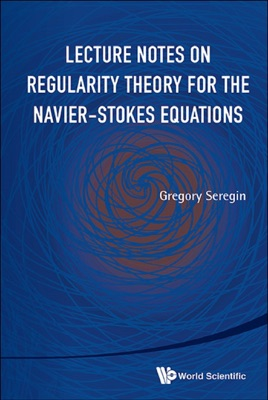• 26,99 €

## Description de l’éditeur

The lecture notes in this book are based on the TCC (Taught Course Centre for graduates) course given by the author in Trinity Terms of 2009–2011 at the Mathematical Institute of Oxford University. It contains more or less an elementary introduction to the mathematical theory of the Navier–Stokes equations as well as the modern regularity theory for them. The latter is developed by means of the classical PDE's theory in the style that is quite typical for St Petersburg's mathematical school of the Navier–Stokes equations.

The global unique solvability (well-posedness) of initial boundary value problems for the Navier–Stokes equations is in fact one of the seven Millennium problems stated by the Clay Mathematical Institute in 2000. It has not been solved yet. However, a deep connection between regularity and well-posedness is known and can be used to attack the above challenging problem. This type of approach is not very well presented in the modern books on the mathematical theory of the Navier–Stokes equations. Together with introduction chapters, the lecture notes will be a self-contained account on the topic from the very basic stuff to the state-of-art in the field.
Contents:PreliminariesLinear Stationary ProblemNon-Linear Stationary ProblemLinear Non-Stationary ProblemNon-Linear Non-Stationary ProblemLocal Regularity Theory for Non-Stationary Navier–Stokes EquationsBehaviour of L3-NormAppendix A: Backward Uniqueness and Unique ContinuationAppendix B: Lemarie-Riesset Local Energy Solutions